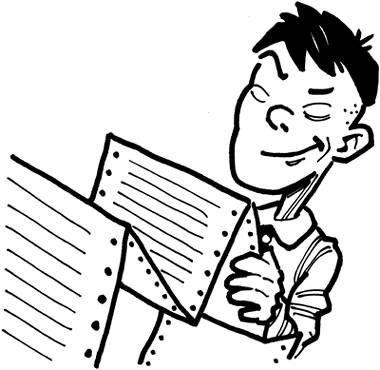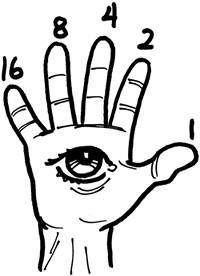# project: know your numbers

we've briefly mentioned the binary and hexadecimal numbering systems before, but we really should give a solid explanation of how both work. when uber-geeks speak in 1337, they sometimes do so using binary or hex code. obviously people cheat; you can easily find web based binary-to-english converters and vice versa but, actually being able to understand this crap really is fun for authentic geeks.

as you should know, a bit is either a one or a zero. a term used less frequently is called a "nibble" which is four bits. take 8 bits and you've got one of the most commonly used terms, a byte. binary can be represented with any number of bits.

so yeah, how does it work? binary is a base 2 number, which is easy to remember because you can only use two digits (1 or 0) and the system is based on powers of 2. before we can count in binary, we need to set up a mental table in our head. when creating our table, we need to read from right to left (like chinese people) rather than left to right as you're probably more accustomed to (unless you are chinese). so, to represent an 8-bit binary number, we need an 8 cell table (counting up from zero).7 6 5 4 3 2 1 0

now, if you take each of those cell numbers to the 2nd power:

2^7, 2^6, 2^5, 2^4, 2^3, 2^2, 2^1, 2^0

we should end up with the following table:

 128 64 32 16 8 4 2 1

start a new row in your table with another 8 cells, and place the bits of your binary number directly below the numbers in the top row. for example, let's say we've got the binary number 11101110; our final table will look like this:

 128 64 32 16 8 4 2 1 1 1 1 0 1 1 1 0

now all you need to do is multiply one number at a time (in the top row) with the number directly below it (you can skip the zeros obviously, as anything multiplied by zero is zero) and after you've multiplied all your numbers, add the results together and you have your decimal representation. in our case:

 128 64 32 16 8 4 2 1 128 +64 +32 +0 +8 +4 +2 +0 = 238

an 8-bit binary number (or byte) can represent the decimal values: 0255 (that's 256 distinct values; always remember the 0). if you remember the site we looked at earlier in the book (www.asciitable.com) then you may have noticed that the table provides 128 different characters, numbered:

0127. considering that every character you could possibly type on an english keyboard is represented in ascii by a number less than 128, a single letter of the alphabet takes less than 1 byte (7 bits) to represent internally with binary.using 5 bits with this numbering system (instead of 8) you can count to 31 using just one hand, or all the way up to 1023 using both hands. imagine if you're looking at the palm of your right hand, your fingers would be numbered with our mental table as 16, 8, 4, 2 and 1; it's all mathematics. if counting to ten looks like this:

00000

00001

00010

00011

00100

00101

00110

00111

01000

01001

01010

what number would your middle finger be if you were flipping somebody off? do the math… i flunked math, btw (i'm a visual-aid, which is why i like programming because you're not just working with numbers but objects). that'sbinary, and as they say "there are 10 types of people in this world, those who understand binary and those who don't."

the hexadecimal system is almost identical (mentally) to binary except that it revolves around 16 rather than 2. since you've only got ten numbers (09) the remaining 6 numbers are substituted by letters of the alphabet. in other words, hexadecimal characters are 0, 1, 2, 3, 4, 5, 6, 7, 8, 9, a, b, c, d, e, and f. a = 10, b = 11, and so on all the way up to 15 (a total of 16 characters). as a reminder that we are working with a hex number, some sort of symbol is usually placed before the number such as \$, #, or 0x (a hexadecimal number will end up looking something like this: 0xa34fb0cc). now we can set up a table similar to our binary one, taking things to the 16th power:

16^7, 16^6, 16^5, 16^4, 16^3, 16^2, 16^1, 16^0

the result:

 268,435,456 16,777,216 1,048,576 65536 4096 256 16 1 A(10) 3 4 F(15) B(11) 0 C(12) C(12)

use the same multiplication as before and there you go. as you can see, hexadecimal is certainly a lot prettier than binary for those larger numbers. you might consider printing yourself a copy of the ascii table for reference.Tapeworm - 1337 Hax or Handbook
ISBN: N/A
EAN: N/A
Year: 2005
Pages: 74